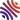## 22 publications1. Computations of general Heun functions from their integral series representations (, and ), In 2021 Days on Diffraction (DD), volume , . [hal-lmpa]
2. Counting walks by their last erased self-avoiding polygons using sieves (), In Discrete Math., volume 344, . [hal-lmpa]
3. Tridiagonalization of systems of coupled linear differential equations with variable coefficients by a Lanczos-like method ( and ), In Linear Algebra and its Applications, volume 624, . [hal-lmpa]
4. On the solutions of linear Volterra equations of the second kind with sum kernels (), In J. Integral Equations Appl., volume 32, . [hal-lmpa]
5. Lanczos-like algorithm for the time-ordered exponential: the $*$-inverse problem ( and ), In Appl. Math., volume 65, . [hal-lmpa]
6. Dynamics of quantum systems driven by time-varying Hamiltonians: Solution for the Bloch-Siegert Hamiltonian and applications to NMR ( and ), In Phys. Rev. Research, American Physical Society, volume 2, . [hal]
7. Computing Optimal Assignments in Linear Time for Approximate Graph Matching (, , and ), In 2019 IEEE International Conference on Data Mining (ICDM), volume , . [hal-lmpa]
8. A general purpose algorithm for counting simple cycles and simple paths of any length (, and ), In Algorithmica, volume 81, . [hal-lmpa]
9. A centrality measure for cycles and subgraphs II ( and ), In Applied Network Science, volume 3, . [hal]
10. Enumerating simple paths from connected induced subgraphs ( and ), In Graphs Combin., volume 34, . [hal]
11. A Hopf algebra for counting cycles (, and ), In Discrete Math., volume 341, . [hal]
12. Cycle-Centrality in Economic and Biological Networks ( and ), In Complex Networks & Their Applications VI (Cherifi, Chantal, Cherifi, Hocine, Karsai, Márton, Musolesi, Mirco, eds.), Springer International Publishing, . [hal]
13. Evaluating balance on social networks from their simple cycles (, and ), In J. Complex Netw., volume 5, . [hal]
14. Algebraic combinatorics on trace monoids: extending number theory to walks on graphs ( and ), In SIAM J. Discrete Math., volume 31, . [hal]
15. On Valid Optimal Assignment Kernels and Applications to Graph Classification (, and ), In Proceedings of the 30th International Conference on Neural Information Processing Systems, Curran Associates Inc., . [hal]
16. Exact inference on Gaussian graphical models of arbitrary topology using path-sums (, , and ), In J. Mach. Learn. Res., volume 17, . [hal]
17. An exact formulation of the time-ordered exponential using path-sums (, , and ), In J. Math. Phys., volume 56, . [hal]
18. Evaluating matrix functions by resummations on graphs: the method of path-sums (, and ), In SIAM J. Matrix Anal. Appl., volume 34, . [hal]
19. Optomechanical control of atoms and molecules (, , and ), In Laser Physics, volume 20, .
20. Long distance beam propagation in colloidal suspensions: comparison between theory and experiment (, , and ), In Optical Trapping and Optical Micromanipulation V (Kishan Dholakia, Gabriel C. Spalding, eds.), SPIE, volume 7038, .
21. Entanglement of a Laguerre-Gaussian cavity mode with a rotating mirror (, and ), In Phys. Rev. A, American Physical Society, volume 77, .
22. Entangling the rovibrational modes of a macroscopic mirror using radiation pressure (, and ), In Phys. Rev. A, American Physical Society, volume 77, .

# Informations

Postes ouverts au LMPA

# À venir

24/05 : Journées Complexes du Nord

30/05 : Journée Arithmétique

21/09 : CJC-MA 2022